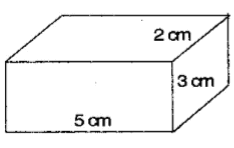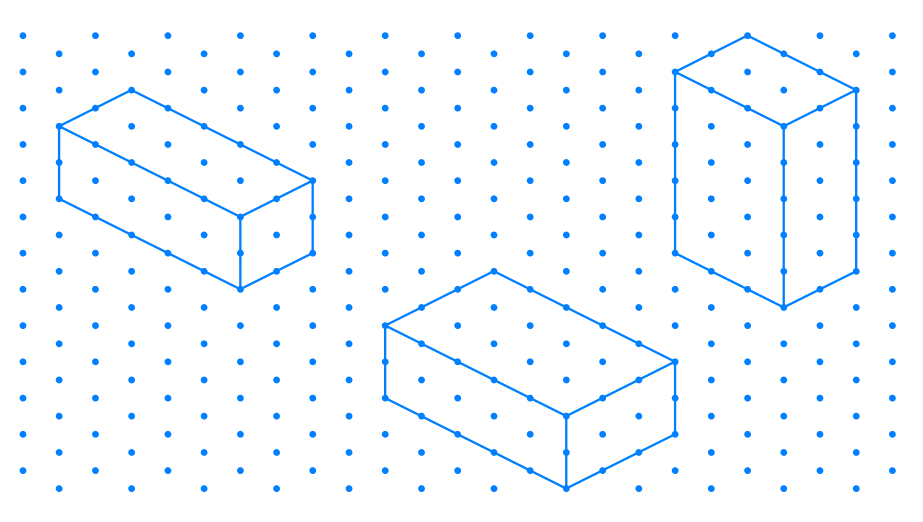# Ex.15.2 Q2 Visualising Solid Shapes - NCERT Maths Class 7

Go back to  'Ex.15.2'

## Question

The dimensions of a cuboid are $$5$$ cm, $$3$$ cm and $$2$$ cm. Draw three different isometric sketches of this cuboid.

Video Solution
Visualizing Solid Shapes
Ex 15.2 | Question 2

## Text Solution

What is known?

Dimensions of a cuboid.

What is unknown?

$$3$$ different isometric sketches of this cuboid.

Reasoning:

Draw $$3$$ rough sketch of cuboid having dimensions of $$5\,\rm{cm}, 2\,\rm{cm}$$ and $$3\, \rm{cm}$$ then draw it on isometric dot paper.

Steps:

The dimensions of cuboid are $$5$$ cm, $$3$$ cm and $$2$$ cm:Three different isometric sketches of given cuboid are below:Learn from the best math teachers and top your exams

• Live one on one classroom and doubt clearing
• Practice worksheets in and after class for conceptual clarity
• Personalized curriculum to keep up with school